blog home

# A linear programming word problem - with a surprise twist!

Subject: Finite Math, Optimization
Courses: M118, MATH135, V118, D117
Posted by: Alex

Today, I thought I'd tackle a problem from one of the most "popular" courses (read "required for almost all majors") at this school - finite math. For those of you who haven't heard of this course before, finite math is actually an amalgam of miscellaneous topics from probability theory, linear algebra, and stats, created by math departments at various universities as a way to introduce college freshmen to rigorous, analytical thinking. It's not a real field of mathematics, in the sense that you won't find any professional mathematicians who specialize in "finite mathematics." However, there are mathematicians who specialize in the topics introduced in this course, such as combinatorics and probability.

One of the topics covered in finite math ("finite", by those in the know) is linear programming. This is basically a fancy term for a constrained optimization problem consisting of linear constraints and a linear objective function. In this blog post, I will tackle the following problem, which I actually found on Yahoo Answers. Despite the title of the problem, it actually does not require calculus (though it could be solved with calculus). To restate the problem:

Example: Kustom Kars does van conversions. The custom conversion requires 15 hours of shop time, 8 hours of painting time, and 1 hour of inspection time. The deluxe conversion requires 12 hours of shop time, 12 hours of painting time, and 1.5 hours of inspection time. There are 90 hours of shop time, 72 hours of painting time, and 10 hours of inspection time available during the coming two weeks. How many conversions of each type should Kustom Kars perform assuming that each custom conversion results in $175 profit and each deluxe conversion results in$225 profit? What is the maximum profit?

This is typical of these sorts of problems, where they present the information in paragraph form - i.e., the dreaded "word problem." The first thing we need to do is extract the relevant data. Notice that there are two types of numbers they give us: time (in hours) and profit (in dollars). One of these types of quantities is going to give us our constraints, while the other will tell us how to formulate the objective (if you don't know what "constraint" and "objective" mean, please take a moment to review your notes/textbook).

I've highlighted the key phrases that will help us distinguish which is which. "Time available" is a dead giveaway that we're looking at information for our constraints. We are constrained by the amount of time available. "Maximum profit," on the other hand, is talking about the objective function - our objective is to maximize profit.

The next step is to identify our variables. This is the part where I think a lot of students get caught up, in linear programming and word problems in general. The key here is to look at the objective function - profit, in this case. Which of the things mentioned in the problem will ultimately be used to calculate our profit? Well, let's take a look at this sentence: "each custom conversion results in $175 profit and each deluxe conversion results in$225 profit..." So, profit depends on the number of custom conversions and the number of deluxe conversions. These, my friends, are our variables.

Let's call the number of custom conversions $x$ and the number of deluxe conversions $y$. Since we know how much profit they earn from each of these, we can write our objective function: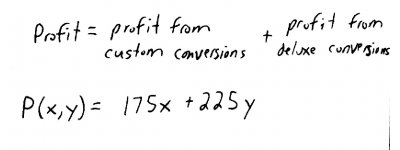So our goal is to maximize $P(x,y)$. Great! Next, we have some constraints. These are given by the 2nd, 3rd, and 4th sentences of the problem: "The custom conversion requires 15 hours of shop time, 8 hours of painting time, and 1 hour of inspection time. The deluxe conversion requires 12 hours of shop time, 12 hours of painting time, and 1.5 hours of inspection time. There are 90 hours of shop time, 72 hours of painting time, and 10 hours of inspection time available..."

The easiest way to handle this data is to create a table: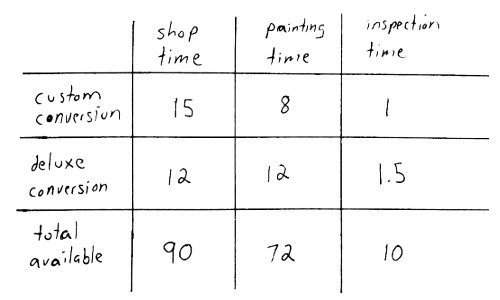From this, we can extract our constraints. First, notice that there are three "ingredients" that go into each type of conversion. Each of these ingredients can be expressed in terms of the number of conversions of each type that the company does: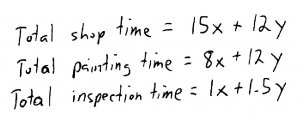Next, notice that there is a limit to the total shop time, painting time, and inspection time (bottom row of the table). This lets us construct the inequalities: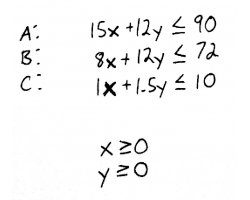I've labeled these $A$, $B$, and $C$. I've also added two more "trivial" (actually, very important) constraints: $x\geq 0$ and $y\geq 0$. These are important because we can't have a negative number of conversions. Between the objective function and these inequalities, we have a fully formed constrained optimization problem. In other words, we've transformed a situation in the real world into the world of math, where we can use everything we know about math to solve the problem, and then bring our answer back into the real world.

To solve this problem, we start by graphing the constraints. To do this, we first convert our inequalities into equalities. These equalities are basically equations of lines. Since lines can be completely defined by two points, all we need to do is find two points for each equation and draw a line between them. The easiest way to find two points is to first set $x=0$ and solve for $y$, and then set $y=0$ and solve for $x$. So, let's do that for each inequality constraint: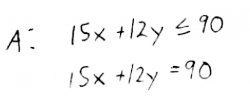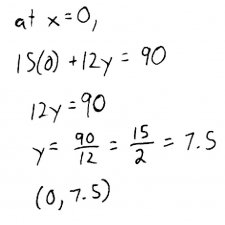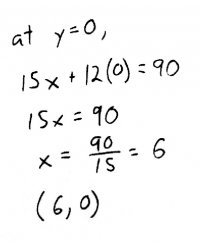So for line $A$, we get the points $(0, 7.5)$ and $(6, 0)$. For $B$, we get: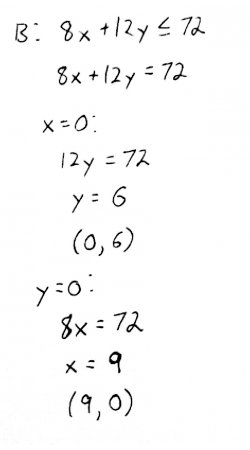And for $C$, we get: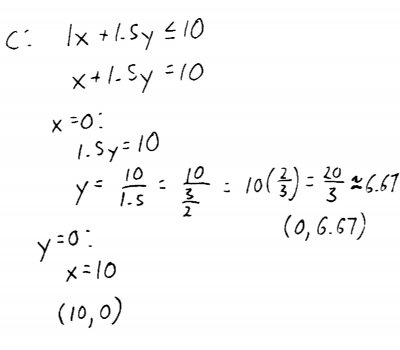Plotting these six points, and drawing lines through the corresponding pairs of points for each constraint, we get this graph: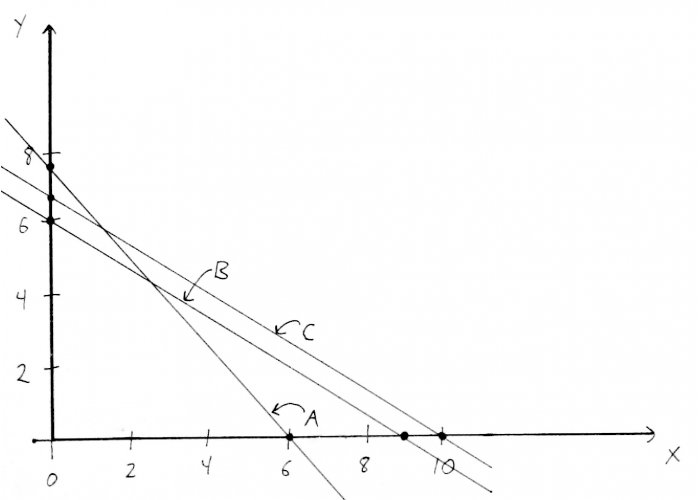Since we're looking for the values of $x$ and $y$ that maximize profit, our solution will be a point on this graph. But, not just any point! It must be a point that obeys our constraints. Which points obey our constraints, you ask? To answer this question, we will identify what's known as the feasible region. Notice that the lines we just drew actually carve up the x-y plane into several pieces (some of which may not be completely bounded!) The feasible region is going to be one of these pieces, in which all points obey our constraints. The easiest way to find the feasible region is by picking a test point from each region, and plugging it into the constraints. If the point satisfies all the constraints, then that region is our feasible region.

We can also hazard a guess that, since all of our constraints involve "less than or equal to," that the feasible region will be the lower left hand section, above the $x$ and $y$ axes. To confirm this, pick the test point $(2, 2)$: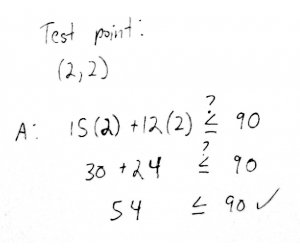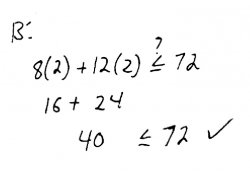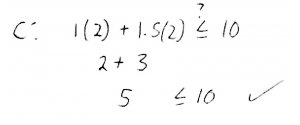Great! The point $(2, 2)$ (in red) satisfies all the constraints, so the region in which it lies is our feasible region. Notice that this region can also be looked at as a polygon (in this case, a quadrilateral). I've labeled the vertices of this polygon $P$, $Q$, $R$, and $S$, for reasons which will become clear in a moment.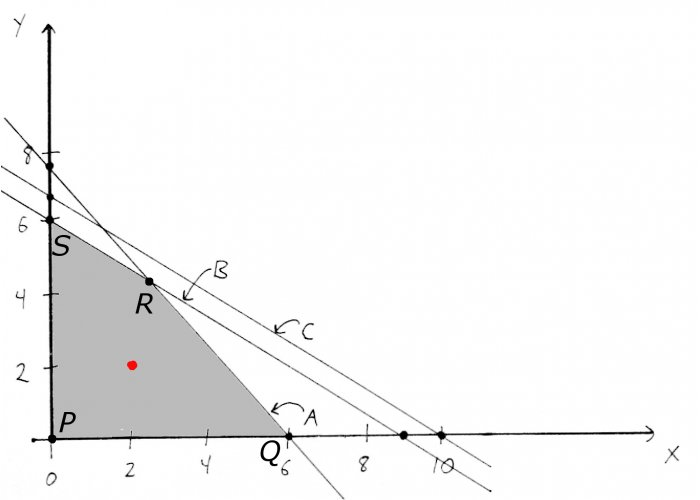Ok, now that we have our feasible region, how do we find the point that corresponds to our optimal solution? Well, we have a theorem called the maximum principle, which for 2D linear programming problems like this, tells us that the optimal solution (if it exists) will lie on one of the vertices (also known as corner points) of the feasible region.

So, all we have to do is to test each of these points in our objective function, and find the point that gives us, in this case, the maximum profit. Well to do this, we need the coordinates of each point. The coordinates of $P$, $Q$, and $S$ are easy: $P$ is the origin, and $Q$ and $S$ are the same points that we used to construct lines $A$ and $B$. But what about point $R$? Well, $R$ is simply the intersection of lines $A$ and $B$. To find the intersection of two lines, recall that we need to solve them as a system of equations: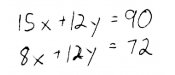The easiest way to solve this is by subtracting the two equations, and then back-substituting: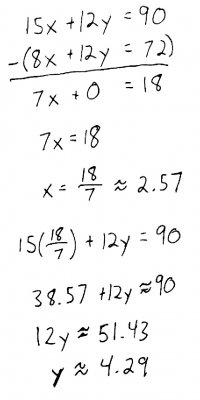Now that we have our four corner points, we can plug them into the objective function and find the profit for each $(x, y)$ pair: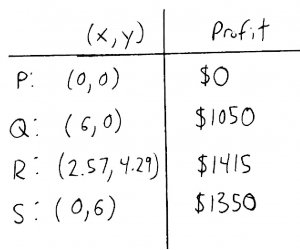Alright, so we just pick point $R$ because that gives us the highest profit, right? No. Be careful. Remember that $x$ and $y$ correspond to real things - the number of custom and deluxe conversions. We can't have 2.57 jobs - or we'd have some very irate customers! We need to round these to whole numbers, such that they still satisfy all of the constraints. We could round to $(2, 4)$, $(3, 5)$, $(3, 4)$, or $(2, 5)$. Well, the only one of these points that won't violate one of our constraints is $(2, 4)$, so we will update R with this point: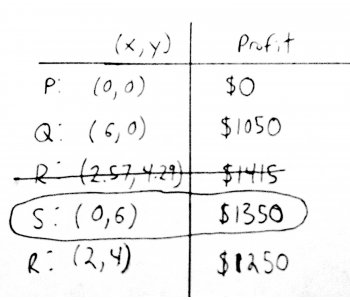Once we round $R$ to the nearest whole number, we need to recompute profit. Now, it's not the optimal point anymore - point $S$ now gives us the highest profit! In a sense, we've introduced another constraint to the problem, namely, that $x$ and $y$ be integers. This type of constraint is actually a topic in of itself, integer programming, which we won't get into today. Suffice it to say, we've found the optimal solution to the problem: Kustom Kars (terrible name by the way), should do 0 custom conversions and 6 deluxe conversions for a profit of $1350. Hope everyone enjoyed that! Update (thanks to reddit user zifyoip): when dealing with integer problems, rounding the non-integer points no longer guarantees us an optimal solution. For this problem it happened to work, but in general the best we can say is that the optimal profit is at least$1350. Thanks!